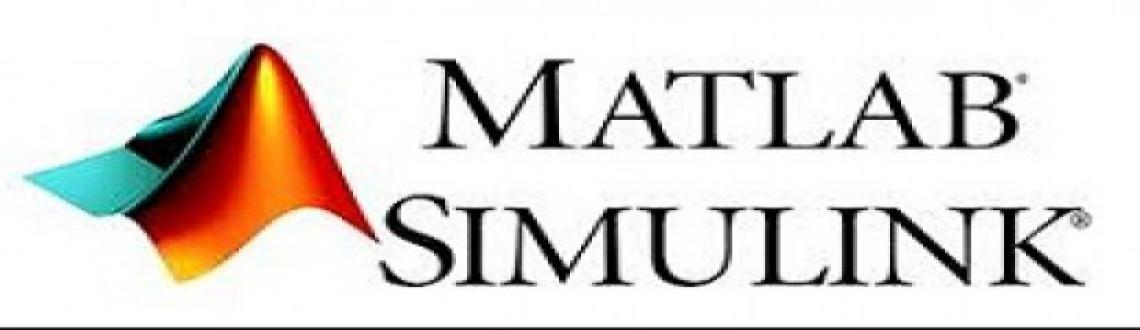# MATLAB Simulink Workshop

• ## MATLAB Simulink Workshop

This workshop aims to make students well-equipped with MATLAB.

Sale Date Ended

INR 1000
Sold Out

Page Views : 206

# MatLab & Simulink Workshop

Program Name - MATLAB & Simulink Workshop
Program Type – Hands on Workshop
Duration – 1 day / 2 day
Registration Process – Fill up the form on the right column of this page and our staff will get in touch with you to guide you through the further registration process.

Introduction:

UPRISE ACADEMY comes up with workshop on MATLAB. This workshop aims to make students well-equipped with MATLAB. The Participants get to know the various nuances of the theory as well as experience on pratical. thus enabling them to link theory and practice.

Program Content

• Introduction to programming .
• Starting with MATLAB.
• Creating Arrays, Mathematical Operations with Arrays .
• Built in MATLAB functions .
• Plotting in MATLAB.
• Symbolic Math .
• Relational operators .
• Programming in MATLAB.
• Functions and Function Files .
• Sparse Arrays, cell arrays and structures .
• Input and output functions .
• Mathematical Modeling and Simulation in MATLAB and SIMULINK .
• Exploring frequently used tool box.
• Introduction to Modeling and Mathematical Modeling .
##### Totorial + Software DVD contents:
1. MATLAB Software.
2. Tutorial on Matlab & Simulink.